28 Rates

# Attenuated total reflectance (ATR)

Attenuated total reflectance or ATR is, along with transmission, one of the most common sampling techniques in Fourier transform infrared (FTIR) spectroscopy. ATR is based on total internal reflection where infrared (IR) light and sample interact only at the point where IR light is reflected. Transmission, on the other hand, is based on IR light passing through the sample.

FTIR spectroscopy is a powerful tool to qualify and quantify organic substances in the solid, liquid, or gaseous state. However, many sampling techniques require pre-treatment of samples in order to obtain high-quality spectra. ATR FTIR spectroscopy allows solid or liquid samples to be measured with only minimal sample preparation.

## Principles of attenuated total reflectance

In ATR FTIR spectroscopy the sample is in contact with the ATR crystal. The IR radiation travels through the crystal and interacts with the sample on the surface in contact with the ATR crystal. Because of the differences in refractive indices of both materials, total internal reflection occurs. This reflection forms the so-called “evanescent wave” which extends into the sample. Based on the sample’s composition, a small part of the infrared light is absorbed when the evanescent wave interacts with the sample, resulting in a slightly attenuated total reflection.

### Total internal reflection

When a propagating wave (e.g. infrared light beam) hits the surface between two optical media which are characterized by two different refractive indices at a certain angle of incidence, the light is totally reflected. This angle is called the critical angle and can be calculated using Snell’s law.

Snell’s law states that the ratio of two refractive indices is equal to the inverse ratio of the angle of incidence and the angle of refraction. For the special case of no refraction, the angle of incidence becomes the so-called critical angle.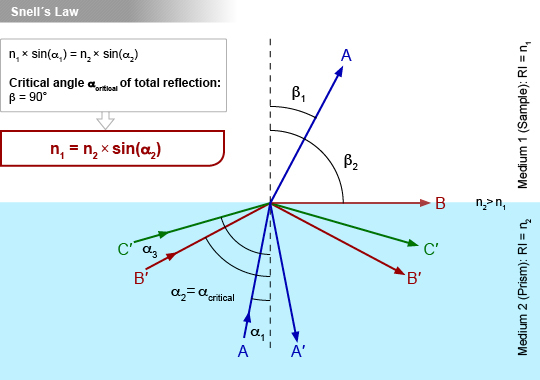Figure 1 Incoming light rays are either totally reflected (α>αcritical) or partly refracted and partly reflected (α<αcritical), depending on their angle.

Many organic samples have mid-IR refractive indices of around 1.5; therefore, the critical angle for ATR crystal materials can be calculated for a refractive index of 1.5.

Table 1 Common materials for ATR crystals; critical angle calculated for n2=1.5; table taken from Griffiths P.R., de Haseth J. A. (2007). Fourier Transform Infrared Spectrometry. New York, USA: John Wiley &Sons.

Cell materialRefractive indexCritical angle (degrees)
Silver chloride AgCl1.9049°
KRS-52.3740°
Zinc selenide (ZnSe)2.4040°
Zinc sulfide (ZnS)2.243°
Germanium (Ge)4.0022°
Silicon (Si)3.4126°
Diamond2.4140°

To obtain total internal reflection, ATR crystal materials must have a higher refractive index than the tested sample material. Because of their high refractive indices, ATR crystals are said to be optically dense whereas typical samples have low refractive indices and therefore are optically rare. The angle of incidence of the IR beam has to match the calculated critical angle for each cell material in order to create total internal reflection.

### Evanescent wave

Total internal reflection does not explain the interaction of the IR beam and sample because the IR beam never leaves the ATR crystal. Instead, the interaction of the IR beam and sample occurs through an evanescent field, often called “evanescent wave”.

Upon total reflection of the incident light at the interface where the crystal touches the sample a small fraction of the light extends into the sample as an evanescent wave. When a wave cannot propagate regularly into the sample, it is concentrated in proximity to the point of total reflection and starts decaying exponentially. The evanescent wave sticks out into the sample.

In areas where the sample is in contact with the evanescent wave specific parts of the IR beam are absorbed based on the sample’s composition. The totally reflected IR light lacks the absorbed parts and thus is attenuated, hence the name “attenuated total reflectance” (ATR).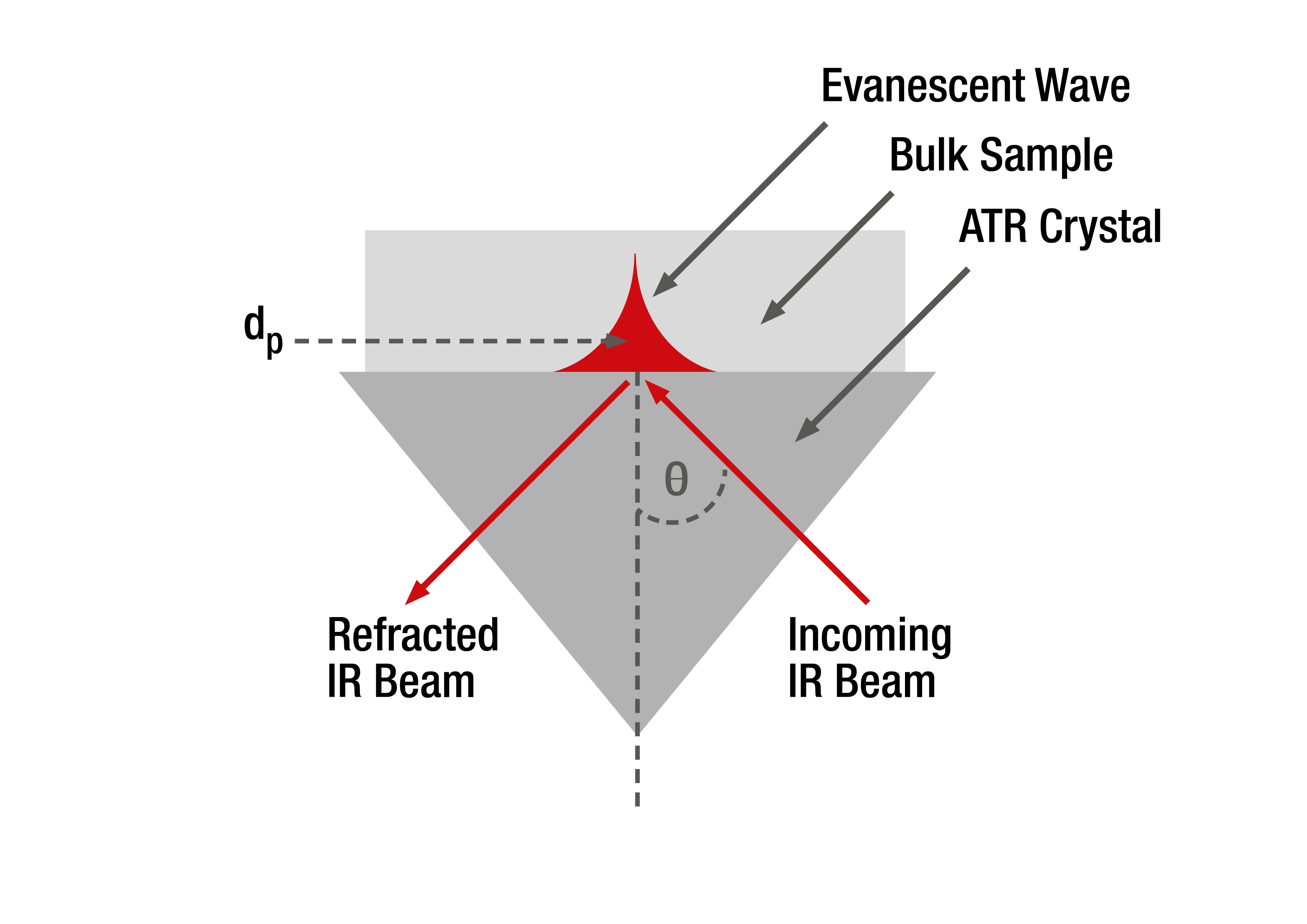Figure 2 Evanescent wave resulting from total internal reflection

### Penetration depth

The penetration depth is a measure of how far the evanescent wave extends into the sample. Its exact value is determined by the wavelength of light, the angle of incidence on the interface between crystal and sample, and the indices of refraction for the ATR crystal and the sample medium. The depth of penetration is defined as the distance to the point at which the evanescent wave’s amplitude has decreased to 1/e (i.e. about 37 %) of its maximum value and can be calculated with the following equation:

Calculation of penetration depth:

λ … wavelength of incident light in vacuum
n1 … refractive index of ATR crystal (dense medium)
n2 … refractive index of sample (rare medium)
ϴ … angle of incidence

According to the above equation the penetration depth for an ATR cell made of zinc selenide, a sample with a refractive index of 1.5 and a wavelength of 10 µm is approximately 2.0 µm. Most organic samples have a refractive index of 1.5; therefore this value is widely used for calculation.

The penetration depth is subjected to changes depending on varying incident wavelengths. Since the IR beam used in FTIR spectroscopy contains a broad range of wavelengths, the penetration depth is different for each wavelength. In regular transmission cells the IR beam passes through the sample; the space where IR beam and sample interact depends only on the length of this transmission cell. The path length is the same for every wavelength. Therefore, absorbance spectra measured with transmission cells are different from spectra measured with ATR cells. In a first approximation this can be accounted for by a multiplication of the ATR spectra with wavenumbers. This is often called a simple ATR correction.

## Attenuated total reflectance in FTIR spectrometers

Many different ATR accessories are available for FTIR spectrometers. They can be divided into ATR cells with a single reflection (one bounce) and cells with multiple reflections (multiple bounce). Depending on the application and the measured samples, different materials are used as the ATR crystal. Typical materials include zinc selenide (ZnSe), germanium (Ge), and diamond.

### Single-bounce ATR cell

In this setup the IR beam and the sample interact only once at a single point of reflection. Modern FTIR spectrometers with sufficiently large signal-to-noise ratios (which is a measure of signal quality) allow for reasonable spectra even with only one bounce. The clear advantage of single-bounce ATR cells is the minimal amount of sample needed for a measurement.

Single-bounce attenuated total reflectance cells are commonly used for solid samples or powders or whenever only small sample volumes of liquids are available. Common single-bounce ATR accessories consist of an ATR crystal with an interface surface area of about 2 mm2 and a clamp which is used to uniformly press solid or powder samples onto the ATR crystal’s surface.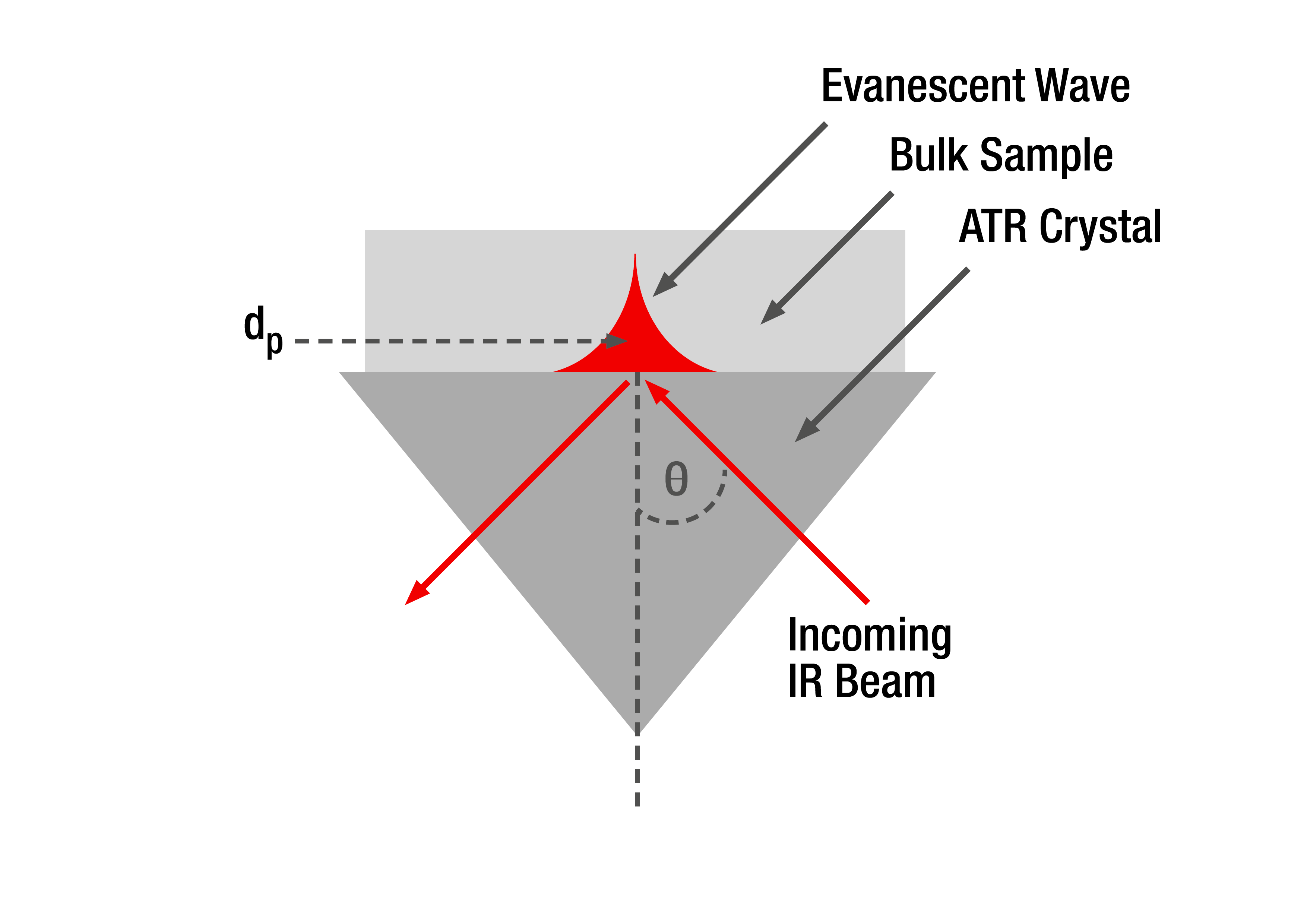Figure 3 Schematic setup of a single bounce ATR cell

### Multiple-bounce ATR cell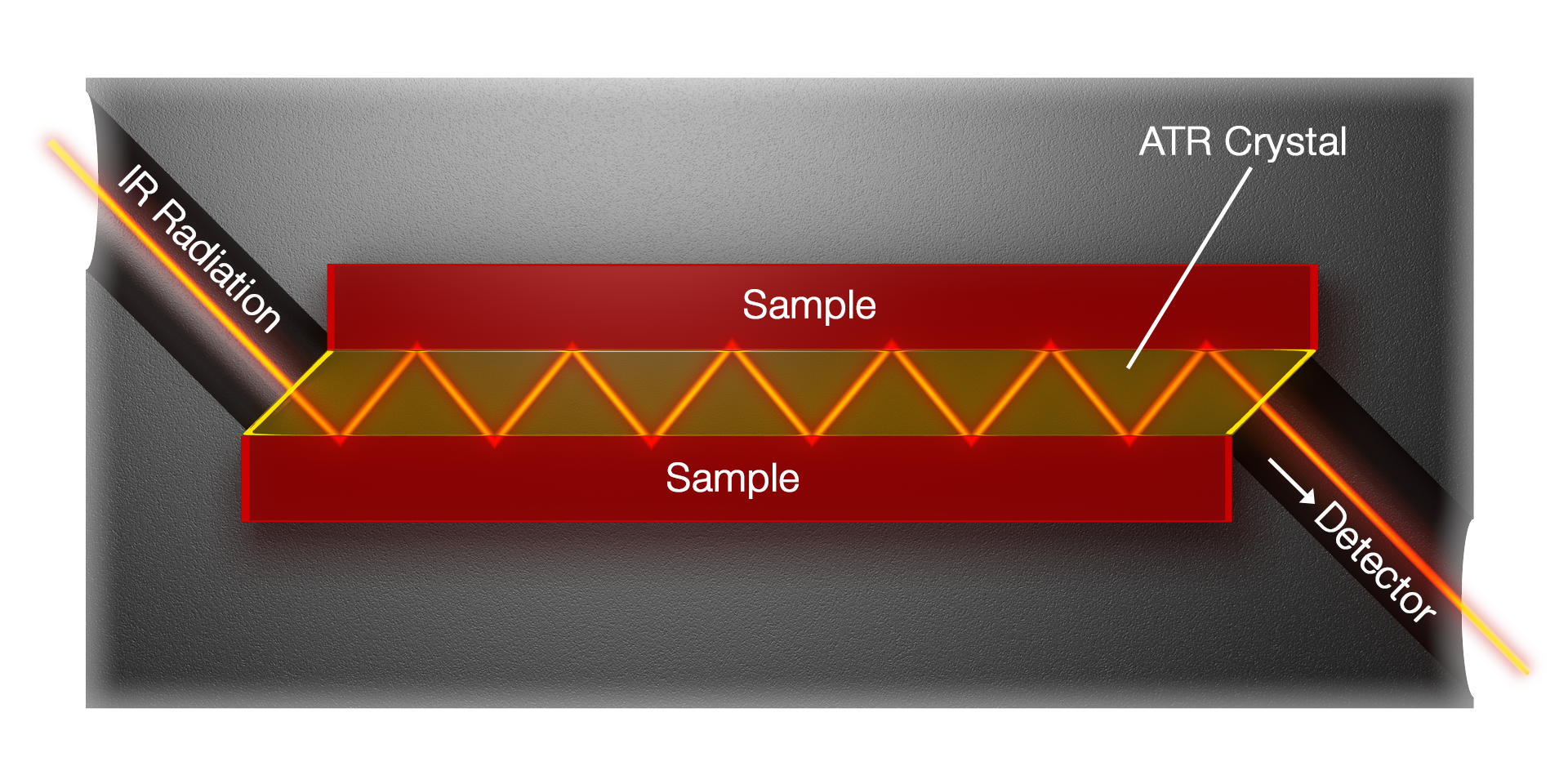Figure 4 Schematic setup of a 12-bounce ATR cell

In multiple-bounce ATR cells the IR beam is reflected multiple times. Each reflection on the ATR crystal’s surface exhibits an evanescent wave which interacts with the sample. Since the interactions are independent of one another the absorptions are additive and the sensitivity of the recorded spectrum can be increased due to significantly higher signal-to-noise ratios.

The attenuated total reflection cell in Lyza 5000 Wine is a 12-bounce ATR cell made of Germanium. The effective penetration depths of those 12 reflections add up to a total (interaction) path length of about 33 μm for organic samples with a refractive index of 1.5.

The graph below shows the change in intensity of a wine spectrum with increasing number of bounces.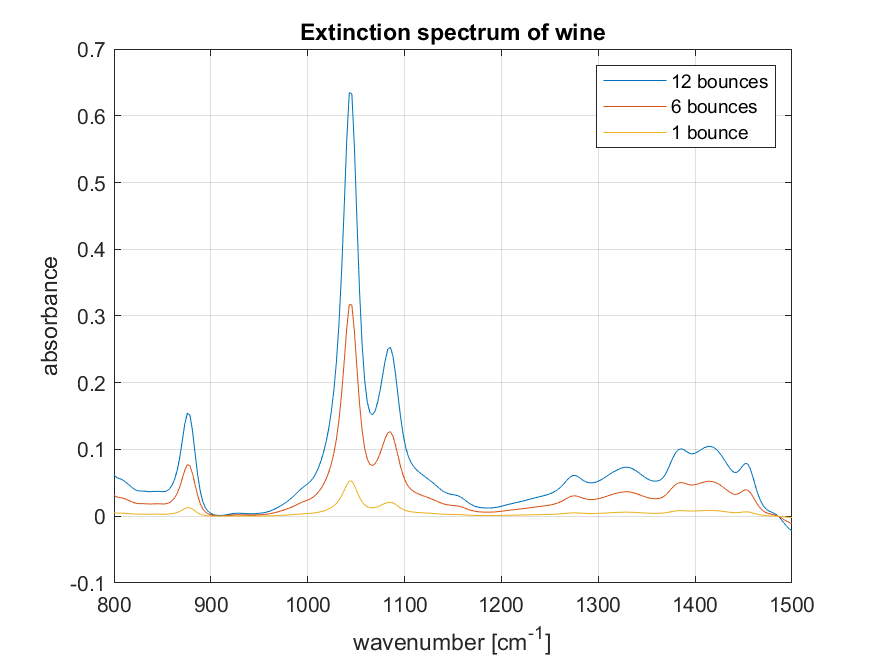Figure 5 Extinction spectrum of wine recorded with different numbers of reflections, overlaid.

## Conclusion

Attenuated total reflectance is a very versatile, easy to use FTIR sampling technique because no or only little sample preparation is required. The interaction of IR light and sample is limited to small sample volumes in direct contact with the ATR crystal; consequently only small sample volumes are needed and ATR techniques are very common. For beverage applications the multiple-bounce ATR cell is the ideal choice because signal intensities can be increased leading to high-quality spectra in short measurement times.